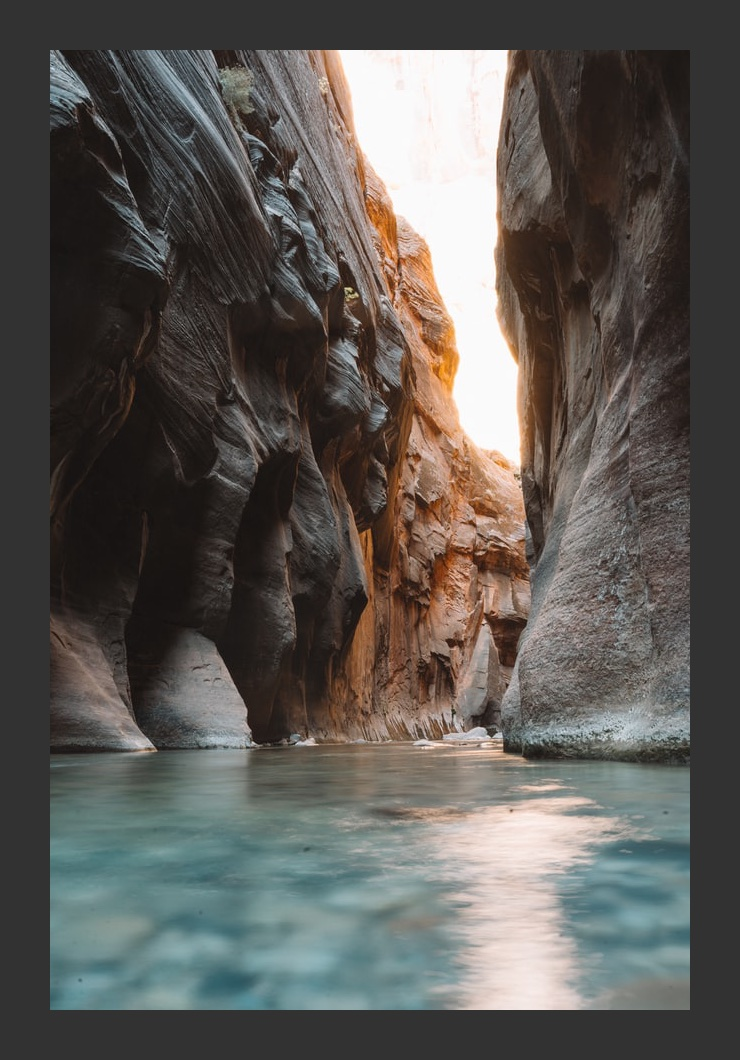Computer Science

# Loops and coordinate transformations

Today we are reviewing concepts to help you better understand some of what we’ve been doing with images.

## Looping in one dimension

First, let’s look at looping in one dimension:

``````from byuimage import Image

image = Image.blank(100, 100)
x = 30
for y in range(image.height):
pixel = image.get_pixel(x, y)
pixel.red = 255
pixel.green = 0
pixel.blue = 0

image.show()``````

We create a blank image that is 100x100 pixels, set `x = 30`, and then loop through just the rows of the image, using the for loop. This creates a thin red line that starts at `x=30` and covers all of the `y` values from 0 to the image height.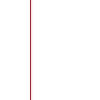Now let’s copy-and-paste that code, and change `x` so that it increments from 30 to 34:

``````x = 30
for y in range(image.height):
pixel = image.get_pixel(x, y)
pixel.red = 255
pixel.green = 0
pixel.blue = 0
x = 31
for y in range(image.height):
pixel = image.get_pixel(x, y)
pixel.red = 255
pixel.green = 0
pixel.blue = 0
x = 32
for y in range(image.height):
pixel = image.get_pixel(x, y)
pixel.red = 255
pixel.green = 0
pixel.blue = 0
x = 33
for y in range(image.height):
pixel = image.get_pixel(x, y)
pixel.red = 255
pixel.green = 0
pixel.blue = 0
x = 34
for y in range(image.height):
pixel = image.get_pixel(x, y)
pixel.red = 255
pixel.green = 0
pixel.blue = 0``````

Now we get a thicker red line that is five pixels wide, covering `x = 30 ... 34` and all the `y` values.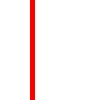## Looping in two dimensions

This is why we need nested for loops! Instead of having to copy-and-paste our code each time, we can instead have one loop to cover the `x` values and one to cover the `y` values:

``````for x in range(30,34):
for y in range(image.height):
pixel = image.get_pixel(x, y)
pixel.red = 255
pixel.green = 0
pixel.blue = 0``````

We will get the same thicker red line:In this case, we will cover the pixels in this order:

• (30, 0)
• (30, 1)
• (30, 2)
• (30, 99)
• (31, 0)
• (31, 1)
• (31, 2)
• (34, 99)

In other words, we are going column-by-column, by fixing `x`, and then doing all the `y` values, and then going to the next `x`.

It makes little difference if we loop over the `y` values first:

``````for y in range(image.height):
for x in range(30,34):
pixel = image.get_pixel(x, y)
pixel.red = 255
pixel.green = 0
pixel.blue = 0``````

We will get the same image, we just cover the pixels in a different order:

• (30, 0)
• (31, 0)
• (32, 0)
• (33, 0)
• (34, 0)
• (30, 1)
• (31, 1)
• (32, 1)
• (34, 99)

In other words, we are going row-by-row, by fixing `y`, and then doing all the `x` values, and then going to the next `y`.

## Writing functions

Anything we have done so far we can put into a function:

``````from byuimage import Image

def draw_bar(image):
for x in range(30, 34):
for y in range(image.height):
pixel = image.get_pixel(x, y)
pixel.red = 255
pixel.green = 0
pixel.blue = 0

my_image = Image.blank(100, 100)
draw_bar(my_image)
my_image.show()``````

We will get the exact same result:A very impressive demo so far!### Generalizing

You can control the height of the bar as well:

``````def draw_bar(image):
for x in range(30, 34):
for y in range(20, 80):
pixel = image.get_pixel(x, y)
pixel.red = 255
pixel.green = 0
pixel.blue = 0``````

This gets us a shorter line: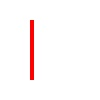Now, take each of those hard-coded values in the for loops and turn them into parameters for the function:

``````from byuimage import Image

def draw_bar(image, x_start, x_end, y_start, y_end):
for x in range(x_start, x_end):
for y in range(y_start, y_end):
pixel = image.get_pixel(x, y)
pixel.red = 255
pixel.green = 0
pixel.blue = 0

my_image = Image.blank(100, 100)
draw_bar(my_image, 30, 60, 40, 70)
my_image.show()``````

Notice that we created four parameters, `x_start`, `x_end`, `y_start`, and `y_end`, one for each of the starting and stopping points used in the `range()` function.

Now we can draw a bar of whatever shape we want, wherever we want in the image!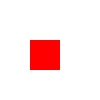It is not a big stretch from here to pass in the colors as well:

``````def draw_bar(image, x_start, x_end, y_start, y_end, red, green, blue):
for x in range(x_start, x_end):
for y in range(y_start, y_end):
pixel = image.get_pixel(x, y)
pixel.red = red
pixel.green = green
pixel.blue = blue

my_image = Image.blank(100, 100)
draw_bar(my_image, 30, 60, 40, 70, 150, 20, 100)
my_image.show()``````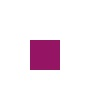## Coordinate transformations

You might prefer to pass in the width and height of the bar instead of having to use coordinates to indicate where the bar stops. You could easily do this by changing the parameters of the function:

``def draw_bar(image, x_start, y_start, width, height, red, green, blue):``

Doing this requires understanding coordinate transformations.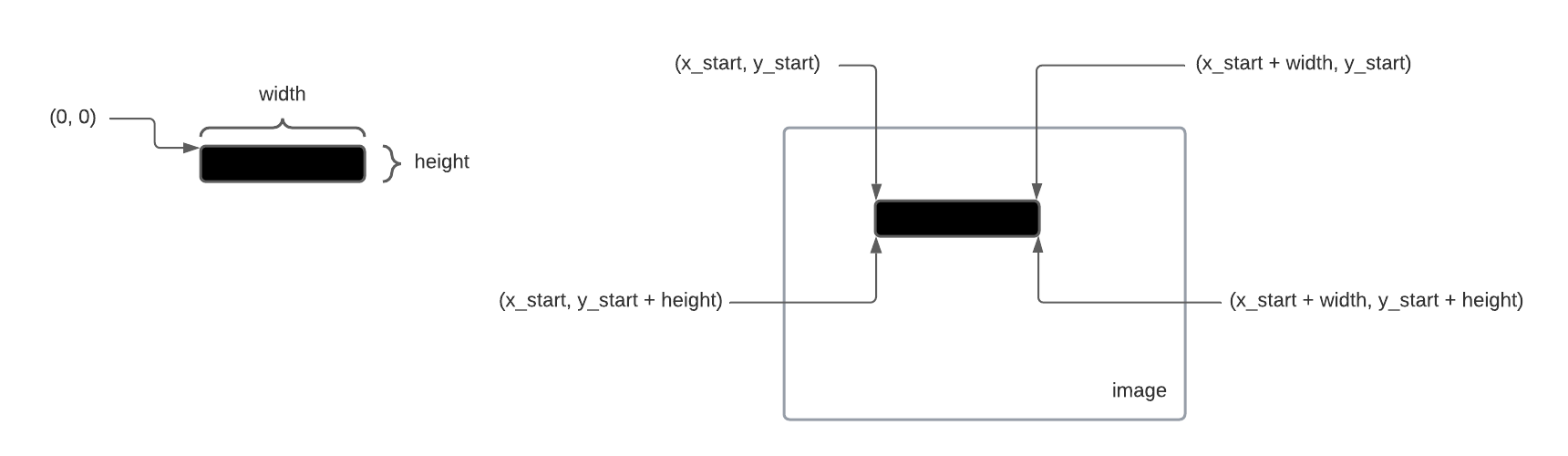We can look at the bar separately, and think of it as starting at a coordinate of `(0, 0)` in the top left corner, with a width and height. That means we can loop over all of its pixels with:

``````for x in range(width):
for y in range(height):``````

But now when we want to get the pixel to draw on the image, we need to transform the coordinates if the bar into the coordinates of the image:

``````for x in range(width):
for y in range(height):
pixel = image.get_pixel(x_start + x, y_start + y)``````

So our complete drawing function would be:

``````def draw_bar(image, x_start, y_start, width, height, red, green, blue):
for y in range(height):
for x in range(width):
pixel = image.get_pixel(x_start + x, y_start + y)
pixel.red = red
pixel.green = green
pixel.blue = blue``````

Alternatively, we can start with the nested for loops looping over pixels in the image directly:

``````for x in range(x_start, x_start + width):
for y in range(y_start, y_start + height):
pixel = image.get_pixel(x, y)``````

This allows us to use `(x, y)` when getting the pixel.

``````def draw_bar(image, x_start, y_start, width, height, red, green, blue):
for x in range(x_start, x_start + width):
for y in range(y_start, y_start + height):
pixel = image.get_pixel(x, y)
pixel.red = red
pixel.green = green
pixel.blue = blue

my_image = Image.blank(100, 100)
draw_bar(my_image, 30, 30, 30, 10, 150, 100, 20)
my_image.show()``````

Either way, we should be able to get the same bar drawn: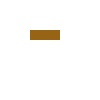We’ve minted a gold bar!## Making borders

Now that we have a function that can draw bars at an arbitrary location, we can use it to draw all the borders of an image:

``````def make_borders(image, thickness, red, green, blue):
# draw top bar
draw_bar(image, 0, 0, image.width, thickness, red, green, blue)
# draw bottom bar
draw_bar(image, 0, image.height - thickness, image.width, thickness, red, green, blue)
# draw left bar
draw_bar(image, 0, 0, thickness, image.height, red, green, blue)
# draw right bar
draw_bar(image, image.width - thickness, 0, thickness, image.height, red, green, blue)

first_image = Image.blank(100, 100)
make_borders(first_image, 30, 255, 0, 0)
second_image = Image.blank(800, 300)
make_borders(second_image, 10, 150, 20, 100)
first_image.show()
second_image.show()``````

Run this code and see what you get!

## Copying images

We use the same concept of coordinate transformation when copying an image.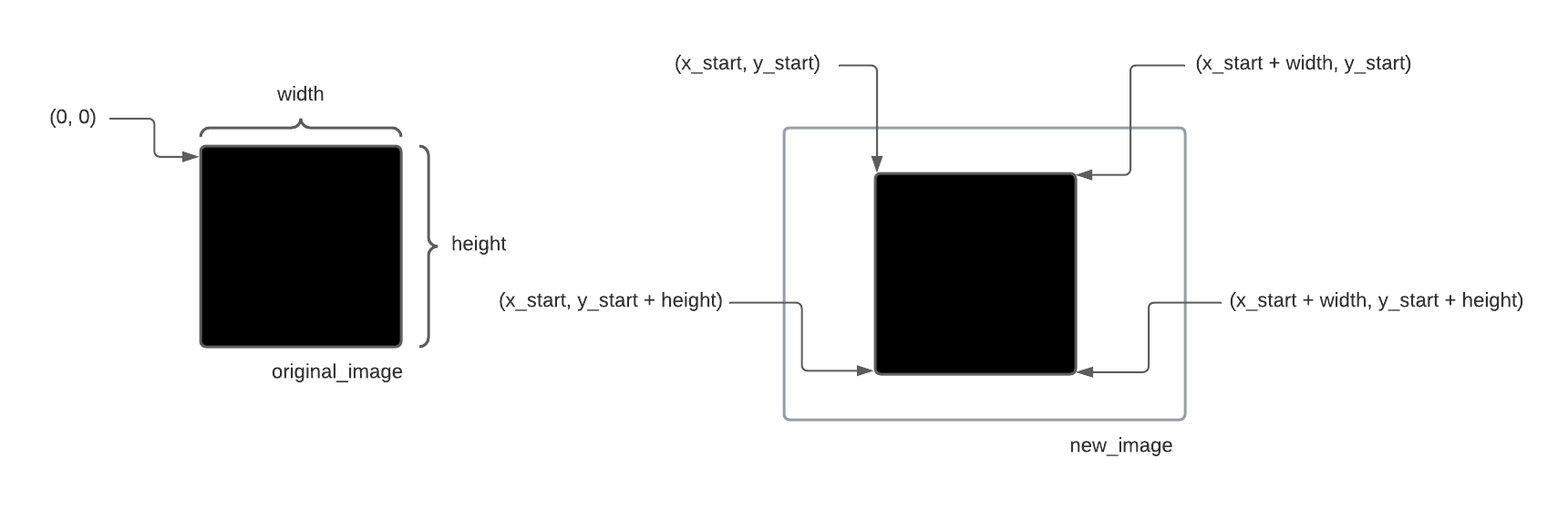Once you write a function to do this:

``def copy_image(new_image, original_image, x_start, y_start):``

then you can use `draw_bar()` and `copy_image()` to make all kinds of new images: